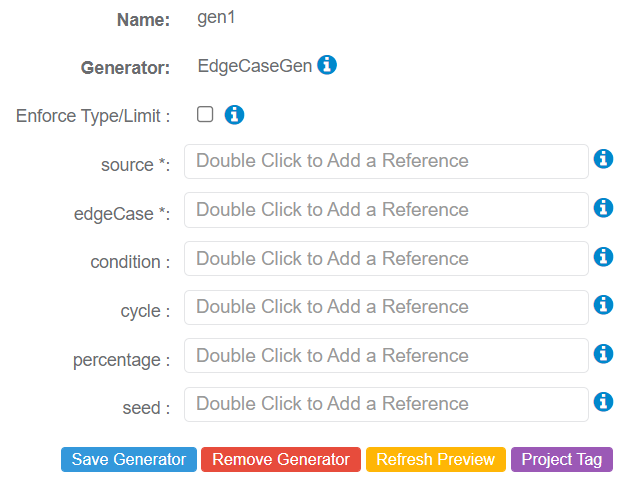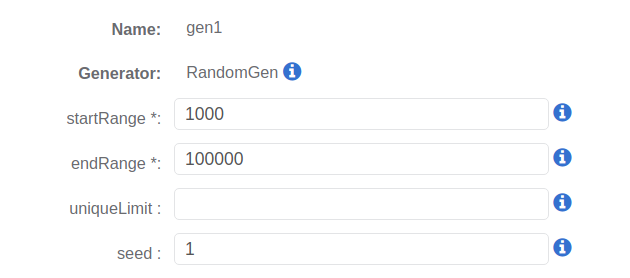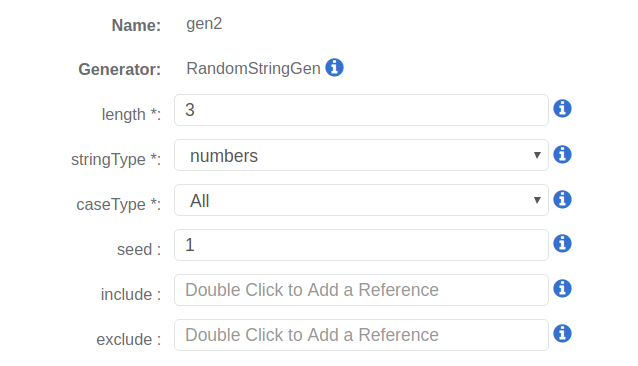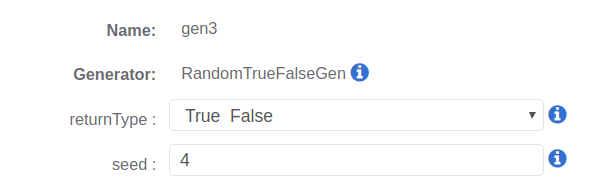# Description

The EdgeCaseGen Generator generates edge case data useful for negative testing and testing edge cases.

## Parameters

The following parameters may be configured for the EdgeCaseGen Generator. Items with an asterisk* are required.

• source* - References a value or Attribute to be returned when the edgeCase condition is false.

• edgeCase* - References a value or Attribute to be returned when the edge case condition is true.

• condition - Reference an Attribute whose Generator will only return a Boolean true or false value. The edgeCase value is return when True, and the source value is returned when False.

• cycle - Defines the number of cycles the source value is returned before the edgeCase value is returned.

• percentage - Defines the percentage of time the edgeCase value is returned as opposed to the source value being returned.

• seed - Sets the seed on the internal random generator that is used when the edge case is being determined by percentage.## Example 1: Create a number between 1000-100,000 when condition is true; otherwise, create a number less than 1000

This example uses four total linked Generators. These three additional Generators are referenced by EdgeCaseGen Generator Parameters:

• RandomGen
• RandomStringGen
• RandomTrueFalseGen

The following are referenced in the EdgeCaseGen Generator configuration:

• source Parameter - References RandomGen (gen1)
• edgeCase Parameter - References RandomStringGen (gen2)
• condition Parameter - References RandomTrueFalseGen (gen3)

##RandomGen Generator Configuration (source Parameter Reference):RandomStringGen Generator Configuration (edgeCase Parameter Reference):RandomTrueFalseGen Generator Configuration (condition Parameter Reference):## Sample Output

gen4 = Result.

if condition is TRUE = result should be 1,000-100,000.

if condition is FALSE = result should be less than 1,000.